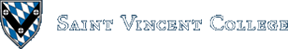Computing & Information Systems    DepartmentSearch CIS Site      Tutorials

## Software Design Using C++

### Recursion

Recursion is a method of solving a problem by solving a simpler version or versions of the original problem (and perhaps doing some additional computations). A recursive solution must break a problem up into one or more simpler versions of the original problem. These might be simpler in that they use a smaller value of a parameter. Also, the chain of recursive calls that gets generated must always end in a stopping case, a case where the answer is directly known so that further recursive function calls are not used.

### The Factorial Function

A favorite example for illustrating recursion is the factorial function. In mathematics, one usually defines the factorial of a non-negative integer n as follows, where n! means the factorial of n.

 `````` 0! = 1 n! = n * (n-1) * (n-2) * ... * 2 * 1 for n > 0 ``````

This easily leads to a normal iterative factorial function. This could be written, for example, as:

 `````` /* Given: n A non-negative integer. Task: To compute the factorial of n. Return: This factorial in the function name. */ long factorial(int n) { int k; long result = 1; for (k = 2; k <= n; k++) result = result * k; return result; } ``````

When this function gets called with a value of 0 or 1 in `n`, the `for` loop gets skipped, so that a value of 1 is returned. Trace the execution of this function for the next few integers to verify that the following factorials are computed correctly:

n factorial(n)
0 1
1 1
2 2
3 6
4 24
5 120
6 720

Note that after the first few lines, the factorials start to get pretty big. That is why we used a `long` to hold the factorials, so that there is less chance of overflow. However, if you use a large enough value of `n`, overflow will still happen. You will start to get meaningless answers for the factorials, such as negative numbers.

Where is the recursion? Thus far we have none. Is there any way of seeing a simpler version of the computation of a factorial inside of the computation of a factorial? Sure. For example, in computing 4! = 4 * 3 * 2 * 1, we see that it is the same as 4 * 3!, where 3! is a simpler factorial computation. A recursive definition of the factorial function can be written as follows:

 `````` 0! = 1 n! = n * (n-1)! for n > 0 ``````

This leads directly to the recursive C++ function found in the test program fact.cpp and copied in below for convenience:

 `````` /* Given: n A non-negative integer. Task: To compute the factorial of n. Return: This factorial in the function name. */ long factorial(int n) { if ((n == 0) || (n == 1)) // stopping cases return 1; else // recursive case return n * factorial(n - 1); } ``````

When the value of `n` is 0 or 1 we have stopping cases, where we directly return the answer. Otherwise, we have the recursive case, where the factorial function calls itself (but with a parameter that is smaller by 1).

How do we trace the execution of a recursive function? One method is to go back to our drawings of the run-time stack. If, for example, we had a test program that called `factorial(3)` we would get a picture of the run-time stack as shown below: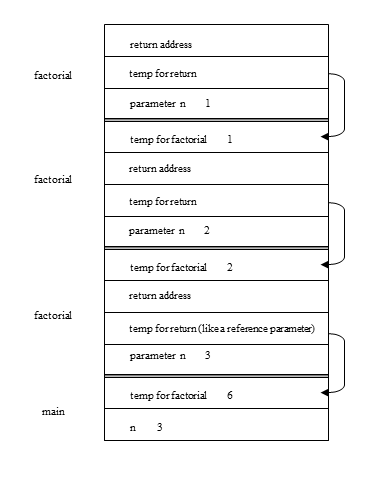Since our function returns an answer in the function name, we assume as usual that the compiler sets this up to operate like a reference parameter, using a pointer to some temporary location for holding the factorial value. The `main` function calls `factorial` so that a stack frame for `factorial` is put on the stack with a value of 3 in the parameter `n`. But that invocation of `factorial` calls factorial again, so another, almost identical, stack frame is put on the stack. This one contains a value of 2 for `n`, however. This invocation calls `factorial` yet another time, so another stack frame for `factorial` is placed on the stack, this time with a value of 1 for `n`.

Thus you can see how the chain of recursive calls results in the placing of successive stack frames for the same function on the run-time stack. This chain ends when we reach a stack frame that corresponds to a stopping case. Since an answer is known for the stopping case, this answer, 1, is sent back to the previous stack frame by following the pointer to the temporary location for the factorial value. The top stack frame is then removed. Then the previous invocation of `factorial` can continue. It finishes the suspended computation of `2 * factorial(1)` using the 1 we just stored. The product, 2, is then stored using the pointer to the temporary location in the previous stack frame. The current stack frame is removed, so that only one stack frame for `factorial` remains. This one finishes its suspended computation of `3 * factorial(2)` as 3 * 2, giving 6. The 6 is stored by following the pointer to the temporary variable for the factorial value out in the stack frame for the `main` function. Finally, the stack frame for `factorial` is removed.

Notice that the stack frames for `factorial` first build up on the stack. When a stopping case is reached, the stack frames start coming off with answers sent back at each step by means of the pointer mechanism. Since the picture is fairly complicated with all of the details of stack frames, one wonders if there might be an easier way to trace a recursive function. One method is to skip the run-time stack and simply show in boxes the original function call and then the code that it generates, followed by any code that that one generates due to a recursive call, etc. Arrows are used to link each call to the code that it generates. A backwards arrow labelled with a value is used to show a result sent back. Here is the simplified drawing: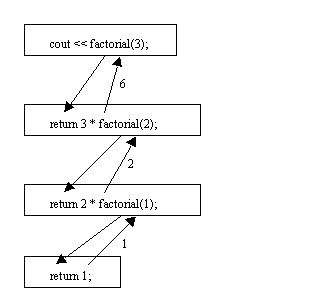That is much simpler to follow. Try tracing a call of `factorial(5)` this way. Also try tracing a call of `factorial(-1)`. What happens? We did not really intend that this function would ever be called with a negative parameter value. Still, nothing prevents us from doing this. Clearly, we will first generate the statement `return -1 * factorial(-2);`, this will generate `return -2 * factorial(-3);`, etc. It looks like it will run forever, since it produces a value of `n` that is 1 smaller each time. However, if you know a little computer architecture, you realize that on most computers successively subtracting 1 eventually yields a positive integer! This is because computer integers have a limited range. When the lowest integer is reached and we subtract 1, we probably get the largest positive integer due to wrap-around. This is the typical result on most computers. Thus the chain of recursion probably ends once we wrap around and decrement `n` to 1, but we will get a nonsense answer. Besides, the mathematical factorial function is not defined for negative numbers. Note that one of our product computations will most likely overflow if we ever even get to the point of computing the products. Another possible result is overflow of the run-time stack. This could occur if we run out of stack space before we hit a stopping case.

### The Fibonacci Function

Another common mathematical function that can be defined recursively is the Fibonacci function. It can be defined as follows:

 `````` fib(0) = 1 fib(1) = 1 fib(n) = fib(n-1) + fib(n-2) for n > 1 ``````

This is a recursive definition, with the first two lines specifying stopping cases. The last line is for the recursive case. It defines fib(n) in terms of fib(n-1) and fib(n-2), two simpler versions of the problem of finding fib(n). One can easily use this definition to figure out that fib(2) = 2, and then that fib(3) = 3, etc. Each new value of the Fibonacci function is the sum of the two previous values. The following table summarizes the first several values of the Fibonacci function. Somewhat like the factorial function, this function starts to get large pretty quickly.

n fib(n)
0 1
1 1
2 2
3 3
4 5
5 8
6 13
7 21
8 34
9 55
10 89

A recursive C++ Fibonacci function can be defined as follows. Note how closely it follows the above mathematical definition.

 `````` /* Given: n A non-negative integer. Task: To find the nth Fibonacci number. Return: This Fibonacci number in the function name. */ long fib(int n) { if ((n == 0) || (n == 1)) // stopping cases return 1; else // recursive case return fib(n - 1) + fib(n - 2); } ``````

Let's draw one of our simplified pictures to trace the operation of this fib function. Let's say that we start by calling fib(4). We show this call in a box with an arrow to the code that it generates. But as you see, the original call generates two more calls. Then the recursive function calls keep splitting out, in the manner of a binary tree, until we reach the stopping cases.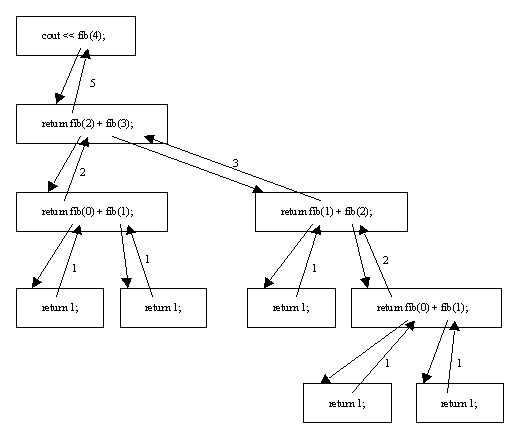Notice how fib(2) gets computed twice! Plus, the stopping cases for the function get called needlessly over and over. There is a lot of wasted effort in this computation of fib(4). The tree of function calls would be even larger and more wasteful if we computed fib(5). This is an instance where a recursive function is the wrong approach to take.

In the complete program, fib.cpp, there is an iterative Fibonacci function that is much more efficient. It uses a simple loop to carry out the computations shown in our table of Fibonacci values above. There is also no needless re-computation of values. So, for the Fibonacci function, iteration is better than recursion. Whenever you see a treeful of recursive function calls, ask yourself if you can compute the same thing with just a linear sequence of actions. If so, try using a loop as a method that may well be faster than the recursion. Note that sometimes an algorithm that gives a treeful of recursive function calls is efficient. This is often true if the tree is very short, so that the stopping cases are reached quickly.

### A Recursive Binary Search

Binary search is often written using recursion, instead of iteration as we did earlier in the array section. The key idea is that when the algorithm decides to search the right or left half of the array, that is a simpler version of the original problem. In the recursive `BinarySearch` function below note how the parameters to the recursive calls are adjusted to specify either the right or left half of the array. See bsearch.cpp for the complete program.

 `````` /* Given: IntArray Array of integers. Low The low index of the range of integers to search. High The top index of the range of integers to search. Target The integer for which to search. Task: To do a recursive binary search for Target in the specified range of IntArray. Return: In the function name, return the index of where Target was found or -1 if it was not found. */ int BinarySearch(IntArrayType IntArray, int Low, int High, int Target) { int Mid, Difference; if (Low > High) return -1; else { Mid = (Low + High) / 2; Difference = IntArray[Mid] - Target; if (Difference == 0) // IntArray[Mid] == Target return Mid; else if (Difference < 0) // IntArray[Mid] < Target return BinarySearch(IntArray, Mid + 1, High, Target); else return BinarySearch(IntArray, Low, Mid - 1, Target); } } ``````

### A Recursive Bubblesort

Bubblesort can also be written using recursion. See bubble.cpp for the details. This one is left to the reader to decipher.

### Quicksort

One of the main reasons for studying recursion is so that one can understand some of the more powerful sorting routines, such as quicksort, which are usually written using recursion. Quicksort is `Theta(n * lg(n))` for average case running time, where `n` is the size of the array. (See the Analysis of Algorithms section for an explanation of the Theta() notation.) This beats the typical bubblesort, insertion sort, and selection sort, all of which are `Theta(n^2)` in the average case. For large values of `n`, the difference in sorting time between quicksort and bubblesort can be substantial. Of course, for really small values of `n`, all of these sort routines are fast and no one cares which one you use.

However, ordinary quicksort has a bad worst case. If the data is already in order (either ascending or descending order) then quicksort degenerates to `Theta(n^2)`. However, data in ascending order is bubblesort's best case, when it is `Theta(n)`. Thus, if the data is already in order (or close to it), bubblesort is likely to beat quicksort. Quicksort also needs extra main memory space, mostly for the runtime stack. Due to the recursive calls that can pile up in the worst case, the amount of extra memory needed is `Theta(n)` in the worst case. (On average, the amount of extra memory needed is `Theta(lg(n))`.)

The code for quicksort and a test program to try it out can be found in the following files.

Let's begin by looking at the second of the files above. The first item of interest is the `inline` function for swapping two integers. Then comes the code for the `QuickSort` function itself, as shown below:

 `````` /* Given: NumArray Array of ints. Lower An index into the array. Upper An index into the array. Task: To quicksort the section of the array from index Lower to Upper. Return: NumArray The sorted array. */ void QuickSort(IntArray NumArray, int Lower, int Upper) { int PivotIndex; if (Lower < Upper) { PivotIndex = Partition(NumArray, Lower, Upper); QuickSort(NumArray, Lower, PivotIndex - 1); // sort left side QuickSort(NumArray, PivotIndex + 1, Upper); // sort right side } } ``````

This function is deceptively simple. This is because it is recursive, calling itself not once, but twice, so that the recursion handles a lot of the work. Second, this is because much of the work is done by the `Partition` function.

Quicksort is sometimes called "sorting by range partitioning" and works approximately as follows: As long as the `Lower` index of the array section to sort is less than the `Upper` index, there is work to be done. (Otherwise we have the stopping case for the recursion.) This work consists of three steps. First, the data in the array is moved around so that the array is partitioned into three sections, with the so-called pivot item as the middle section, items less than or equal to the pivot in the first section, and items greater than the pivot in the third section. This gets the data somewhat in the right vicinity of where it should go once the array is finally sorted. Then the first and third sections are sorted (recursively). At that point, the whole array is in order.

In order to trace how things work, we also need to look at the details of the `Partition` function. Although there are various ways to write such a function, here is our version:

 `````` int Partition(IntArray NumArray, int Lower, int Upper) { int Pivot, Left, Right; Pivot = NumArray[Lower]; Left = Lower; Right = Upper; while (Left < Right) { // scan from left, skipping items that belong there while ((NumArray[Left] <= Pivot) && (Left < Upper)) Left++; // scan from right, skipping items that belong there while (NumArray[Right] > Pivot) Right--; if (Left < Right) Swap(NumArray[Left], NumArray[Right]); } NumArray[Lower] = NumArray[Right]; NumArray[Right] = Pivot; return Right; // return the pivot index } ``````

Let's look at `QuickSort` and `Partition` using pictures. Suppose that we start with the following array of 7 items. `Lower` would be 0 and `Upper` would be 6 in the call of function `QuickSort`.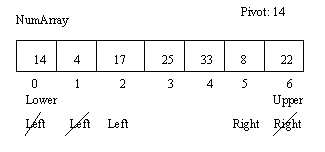`QuickSort`, of course, calls `Partition`. As shown in the above drawing, `Pivot` gets the value of the first item in the array section, 14 in this case. `Left` starts at 0 and `Right` starts at 6. `Partition` then moves `Left` toward the right as long as the item at this index is where it belongs (that is, is smaller or equal to the `Pivot`) and as long as `Left` isn't off the right end of the array. Then `Right` is moved toward the left as long as the item at this index is where it belongs (that is, is greater than the `Pivot`). Then, since `Left < Right`, we swap the items at indices `Left` and `Right`. Here the 17 and 8 get swapped, as shown in the next drawing.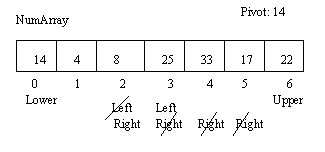The above drawing also shows that when we go around in the outer loop of the `Partition` function, the loop body is executed again with the result that `Left` and `Right` get moved as shown over items that are already in place. Note that the `Right` index has now moved to the left of the `Left` index! Now when we go around in the outer loop and try the `while` condition the loop quits, as `Left < Right` is false. Partition now cleans up by copying `NumArray[Right]` into `NumArray[Lower]`, that is, the 8 is copied into the array slot at index 0. Then the `Pivot` is copied into the array at index `Right`, that is, 14 is copied into the array slot at index 2. Finally, `Partition` returns the `Right` index value, 2, in its function name. The following picture tries to summarize these changes: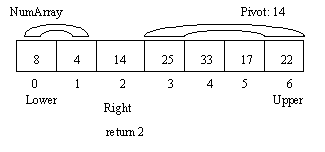Note that the small items are to the left of the pivot, 14, while the large items are to the right. That is what partitioning is supposed to achieve. Now the `QuickSort` function calls itself using the array, 0, and 1 as the parameters, in effect asking that the array section from index 0 to index 1 be sorted. Finally, it calls itself using the array, 3, and 6 as the parameters, asking that the array section from index 3 to index 6 be sorted. Assuming that those recursive calls work OK, the array is now completely sorted (in ascending order).

Of course, each of these recursive calls to `QuickSort` usually generates its own call of `Partition` as well as two new recursive calls to `QuickSort`. However, whenever a recursive call of `QuickSort` amounts to an attempt to sort an empty or 1 item array section, we have a stopping case. Such a recursive call simply returns without doing any work (thanks to the `if` test).

Why does quicksort work so well? It has to do with the way partitioning typically divides each array section into two roughly equal-sized sections to be recursively sorted. The process is reminiscent of binary search in a way, and gives recursive quicksort calls that are nested about `lg(n)` deep, just as binary search had about `lg(n)` probes of the array. Imagine drawing a new picture of the array for each level of nesting, showing the partitioning in effect at that point. You would have about `lg(n)` such pictures. Since the number of comparisons done by the calls to partition for each such picture is `Theta(n)`, we would normally expect Theta(n * lg(n)) efficiency for quicksort. Consult a good data structures book for further details.

The worst case for quicksort is when the data is already in order (ascending order or descending order). This is because the pivot then does not evenly split the array into two halves, but instead splits it into an empty array section and a section with one less than the previous number of items. So, the pattern is much more like that of sequential search than binary search in this regard. This particular problem can be reduced by choosing as pivot the median of the three values at Lower, Upper, and the (approximate) midpoint.

Quicksort can be improved by having another sort used once the recursive calls generate short array sections to be sorted. For example, selection sort might be used on all array sections of less than fifty items.

### Mergesort

Mergesort is another recursive sort algorithm. It is based on finding the midpoint of the array, recursively sorting the two halves, and then merging the halves into one large sorted array. The nice thing about mergesort is that it always has `Theta(n * lg(n))` running time. It has no bad worst case (such as the one that quicksort has where it degenerates to `Theta(n^2)`).

It turns out that the best possible average case and worst case running time efficiency for a sort based on key comparisons is `Theta(n * lg(n))`. Some people call this the Fundamental Theorem of Sorting. One consequence is that it is impossible to have a general sort routine based on key comparisons that is `Theta(n)` on average. Of course, some sort routines are `Theta(n)` in special cases, such as bubblesort when the data is already in order.

Note that a key comparison is when we check two items in the array to see if one is less than another. This means that the entire keys are checked, not just parts of the keys. For example, if we have an array of employee records where the key field is a 5-digit ID number, a key comparison has to check the entire ID of one employee against the entire ID of another employee. (There are sort routines that compare individual digits of the respective keys. They do not fall under this theorem.)

The main thing to note is that quicksort and mergesort achieve this best possible order of magnitude for running time efficiency in the average case. Mergesort even attains it in the worst case. Our version of mergesort does require a temporary array. Thus it needs `Theta(n)` extra main memory space. This is worse than quicksort, which needs on average `Theta(lg(n))` stack space (`Theta(n)` in the worst case), but is not too bad. The following files contain an implementation of mergesort together with a test program.

The main `MergeSort` routine is shown below. The parameters are the array itself, plus the `Lower` and `Upper` indices of the section to be sorted. If `Lower < Upper` we have at least two items in the array section, so that some work has to be done. If not, the section is trivially sorted already.

 `````` void MergeSort(IntArray NumArray, int Lower, int Upper) { IntArray TempArray; int k, Mid; if (Lower < Upper) // if not we have a stopping case { Mid = (Lower + Upper - 1) / 2; MergeSort(NumArray, Lower, Mid); // recursive call MergeSort(NumArray, Mid + 1, Upper); // recursive call // Merge the two sorted pieces together: Merge(TempArray, NumArray, NumArray, Lower, Upper, Lower, Mid, Mid + 1, Upper); // Copy the data back into NumArray: for (k = Lower; k <= Upper; k++) NumArray[k] = TempArray[k]; } } ``````

The overall steps are to find a midpoint `Mid`, to mergesort the two halves of the array, one from `Lower` to `Mid` and the other from `Mid + 1` to `Upper`. These are the recursive calls. Then a `Merge` function is used to merge the two sections together into one large sorted section in `TempArray`. The original array cannot be used as the destination of the data because we would be in danger of overwriting some of the data before we had used it! Finally, the data is copied from `TempArray` to the original array `NumArray`.

Let's look at the mergesort algorithm by using the example pictured below. We start with an array of 7 items and find that the midpoint is 2 using the formula given in the code. (Note that this is deliberately slightly left of the real midpoint. That is because the dividing line between the two sections to sort is between `Mid` and `Mid + 1`.)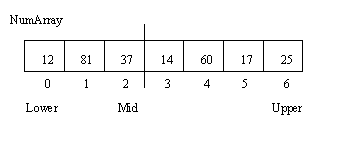Next, the two recursive calls, which amount to `MergeSort(NumArray, 0, 2)` and `MergeSort(NumArray, 3, 6)`, are used to sort the two halves of the array. This gives the following picture.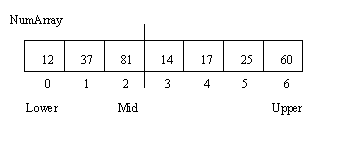Then the data from the two halves is merged together into the temporary array. (The details of the function that handles this merge will be shown later.) This yields the following picture: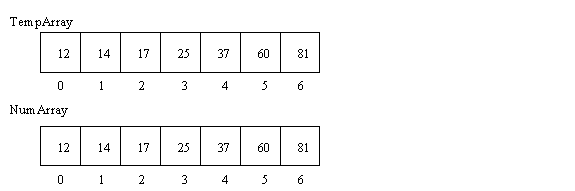The final step, also shown in the last picture above, is simply to copy the data from the temporary array into the original array. (There is a fancier version of mergesort that can reduce the amount of this wasteful copying. Since the fancier algorithm, known as Insort/Outsort, is somewhat more complex, it is not shown here.)

This is the end of the algorithm, though we have skimmed over a number of things, such as the merge routine. Also, the two recursive calls to `MergeSort` each give rise to two more recursive calls to `MergeSort`, etc. until we reach calls that amount to sorting array sections each with only 1 item. These are the stopping cases.

Now let's look at how the `Merge` function works.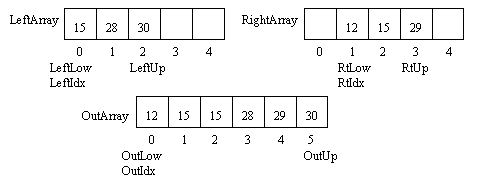See if you can trace how our merge routine copies one number at a time from the two initial sorted array sections into the longer sorted array section.

Back to the main page for Software Design Using C++

Author: Br. David Carlson with contributions by Br. Isidore Minerd
Last updated: April 12, 2016
Disclaimer